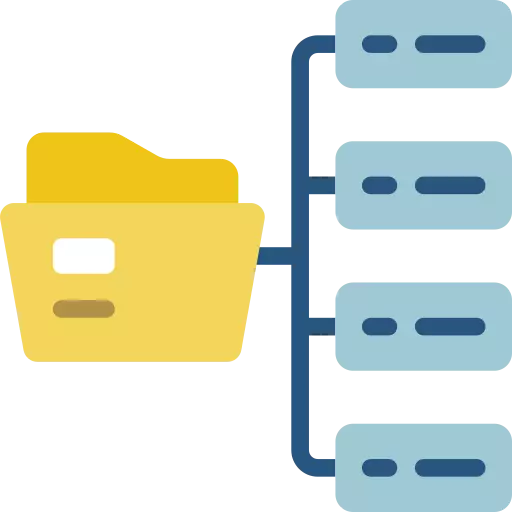# Data Structures and Algorithms In Python## Data Structures and Algorithms In Python

Data Structures and Algorithms In Python is curated in a way to let students learn DSA In Python from the very basics. Look no further than PrepInsta’s Data Structures and Algorithms In Python Tutorial! Whether you’re a computer science enthusiast, a coding novice, or an experienced programmer looking to expand your skill set, this comprehensive course has been designed with you in mind.

Our tutorial takes you on a step-by-step exploration of DSA, starting with the fundamentals of coding and gradually delving into the intricacies of this essential field. Whether you’re new to programming or an experienced coder seeking to add Python to your repertoire, our course has something valuable to offer.

## Time Complexity

Time complexity in Data Structures and Algorithms (DSA) In Python refers to the measure of how the running time of an algorithm grows as the input size increases.## Recursion

Recursion in Data Structures and Algorithms (DSA) with Python is a programming technique where a function calls itself to solve a problem.

• In Python, it’s essential to have a base case to prevent infinite recursion. Recursion is commonly used in solving problems like factorials, Fibonacci sequences, and tree traversals.

## Searching and Sorting

Searching and sorting are fundamental operations in Data Structures and Algorithms (DSA) with Python. Searching involves finding a specific element within a collection of data, using techniques like linear or binary search.

• Sorting arranges data in a specific order, such as ascending or descending, using algorithms like quicksort or mergesort. Efficient searching and sorting are crucial for optimizing data retrieval and organization in various applications.## Stacks

In DSA with Python, a stack is a linear data structure that follows the Last-In-First-Out (LIFO) principle.

• It’s like a stack of plates—items are added and removed from the top. Stacks are used for managing function calls, tracking history, and more.

## Queues

In DSA with Python, a queue is a linear data structure following the First-In-First-Out (FIFO) principle.

• Elements are added at the rear and removed from the front. Queues are used for tasks like scheduling and managing data waiting to be processed.In Python, you can implement a linked list data structure using classes.

• A linked list consists of nodes, where each node has two parts: data and a reference to the next node in the list.

## Trees

Trees are hierarchical data structures that consist of nodes connected by edges. They are used to represent hierarchical relationships, such as organizational structures, file systems, and more.

• One of the most common types of trees is the binary tree, but there are various other types like binary search trees, AVL trees, B-trees, and more.## Binary Search Tree (BST)

A Binary Search Tree (BST) is a widely used data structure in computer science and programming. It is a type of binary tree with a specific property that makes it particularly useful for searching, sorting, and efficient data retrieval.

• A BST is organized in a hierarchical manner, and each node in the tree has a value, a left subtree, and a right subtree.

## Hashing

Hashing in Python is a fundamental concept used for data storage, retrieval, and security. It involves mapping data of arbitrary size to fixed-size values (hash codes) using a hash function.

• This process allows for efficient data retrieval and is commonly used in data structures like dictionaries and hash tables.## Graphs

Graphs are data structures used to represent relationships between objects. They consist of nodes (vertices) connected by edges (links). Libraries like NetworkX and Matplotlib enable graph creation, visualization, and analysis.

•  Graphs are versatile and useful for modeling various systems, such as social networks, transportation networks, and more, facilitating complex data analysis and problem-solving.
• Introduction to Graphs In python
• Importance of Graphs as Data Structures
• Graph Terminology
• Graph implementation
• DFS
• BFS
• Path finding algorithms
• Union Find Problem
• Minimum Spanning Trees
• Detecting Cycles in MST
• Weighted and Directed Graphs
• Cycle Detection in Graphs
• Kruskal’s algorithm
• Prim’s Algorithm
• Dijkstra’s algorithm

## Priority Queues & Heaps

In Python, a priority queue is a data structure that allows you to store elements with associated priorities and efficiently retrieve the element with the highest (or lowest) priority.

• Priority queues are often implemented using a data structure called a heap.## Tries

A trie is a tree structure where each node represents a character, and paths from the root to a node form a string. The root node usually represents an empty string.

• Every node in a trie can have multiple child nodes, each corresponding to a different character.

## Bit Manipulation & Modulo Arithmetic

Bit manipulation involves working with the individual bits (0 or 1) of binary representations of numbers.

• In Python, you can manipulate bits using binary operators like & (AND), | (OR), ^ (XOR), << (left shift), and >> (right shift).